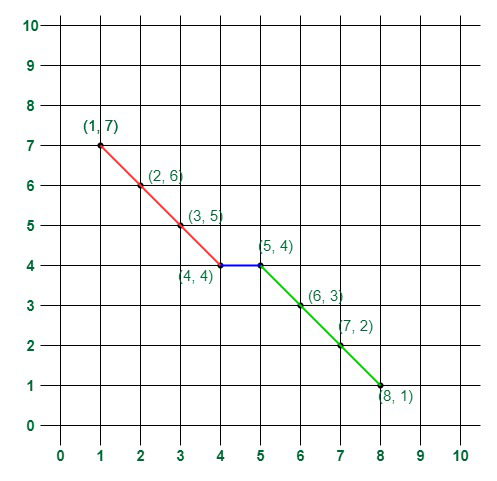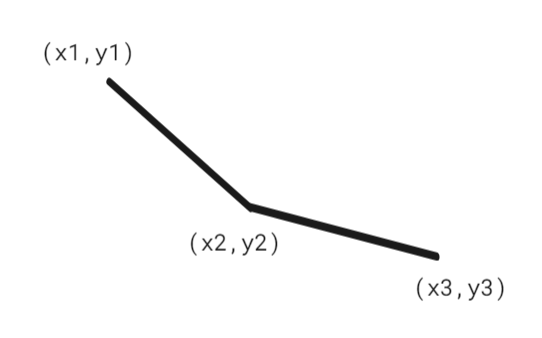GeeksforGeeks App
Open AppBrowser
Continue

# Minimum number of Straight Lines to connect all the given Points

Given 2d integer array arr[][] containing N coordinates each of type {X, Y}, the task is to find the minimum number of straight lines required to connect all the points of the array.

Examples:

Input: arr[][] = {{1, 7}, {2, 6}, {3, 5}, {4, 4}, {5, 4}, {6, 3}, {7, 2}, {8, 1}}
Output: 3
Explanation: The diagram represents the input points and the minimum straight lines required.
The following 3 lines can be drawn to represent the line chart:
=> Line 1 (in red) from (1, 7) to (4, 4) passing through (1, 7), (2, 6), (3, 5), and (4, 4).
=> Line 2 (in blue) from (4, 4) to (5, 4).
=> Line 3 (in green) from (5, 4) to (8, 1) passing through (5, 4), (6, 3), (7, 2), and (8, 1).
It can be shown that it is not possible to represent the line chart using less than 3 lines.Minimum lines to connect the points of the example

Input: arr[][] = {{3, 4}, {1, 2}, {7, 8}, {2, 3}}
Output: 1
Explanation: A single line passing through all the points is enough to connect them

Approach: The problem can be solved based on the following geometrical idea:

If the points are sorted according to their X axis value then to calculate the number of lines check for three consecutive points.
If the slope of lines formed by first two points and the last two points are same then they can be connected by a single line.
Otherwise, we will need two different lines.

To calculate the slope we will use the formulae:
=> slope 1 = (y2 – y1) / (x2 – x1)
=> slope 2 = (y3 – y2) / (x3 – x2)

If slope1 = slope2
(y2 – y1) / (x2 – x1) = (y3 – y2) / (x3 – x2)
(y3 – y2) * (x2 – x1) = (y2 – y1) * (x3 – x2).Lines formed by consecutive 3 points

Follow the steps mentioned below to implement the idea:

• Firstly sort the array based on the value of the X coordinate.
• Traverse the array from i = 0 to N-2:
• If the above condition of slope1 = slope2 is satisfied then continue without incrementing the count of lines.
• Otherwise, increment the count of straight lines required.
• Return the final count of straight lines as the answer.

Below is the implementation of the above approach:

## C++

 `// C++ code to implement the approach` `#include ``using` `namespace` `std;` `// Function to find minimum number of lines``int` `minimumLines(vector >& arr)``{``    ``int` `n = arr.size();` `    ``// Base case when there is only one point,``    ``// then min lines = 0``    ``if` `(n == 1)``        ``return` `0;` `    ``// Sorting in ascending order of X coordinate``    ``sort(arr.begin(), arr.end());` `    ``int` `numoflines = 1;` `    ``// Traverse through points and check``    ``// whether the slopes matches or not.``    ``// If they does not match``    ``// increment the count of lines``    ``for` `(``int` `i = 2; i < n; i++) {``        ``int` `x1 = arr[i];``        ``int` `x2 = arr[i - 1];``        ``int` `x3 = arr[i - 2];``        ``int` `y1 = arr[i];``        ``int` `y2 = arr[i - 1];``        ``int` `y3 = arr[i - 2];``        ``int` `slope1 = (y3 - y2) * (x2 - x1);``        ``int` `slope2 = (y2 - y1) * (x3 - x2);` `        ``if` `(slope1 != slope2)``            ``numoflines++;``    ``}` `    ``// Return the num of lines``    ``return` `numoflines;``}` `// Driver Code``int` `main()``{``    ``vector > vect{ { 1, 7 }, { 2, 6 }, { 3, 5 }, { 4, 4 }, { 5, 4 }, { 6, 3 }, { 7, 2 }, { 8, 1 } };` `    ``// Function call``    ``cout << minimumLines(vect);``    ``return` `0;``}`

## Java

 `// Java code to implement the approach``import` `java.io.*;``import` `java.util.*;` `class` `GFG {``    ``// Function to find minimum number of lines``    ``public` `static` `int` `minimumLines(``int` `arr[][])``    ``{``        ``int` `n = arr.length;` `        ``// Base case when there is only one point,``        ``// then min lines = 0``        ``if` `(n == ``1``)``            ``return` `0``;` `        ``// Sorting in ascending order of X coordinate``        ``Arrays.sort(arr,``                    ``(a, b) -> Integer.compare(a[``0``], b[``0``]));` `        ``int` `numoflines = ``1``;` `        ``// Traverse through points and check``        ``// whether the slopes matches or not.``        ``// If they does not match``        ``// increment the count of lines``        ``for` `(``int` `i = ``2``; i < n; i++) {``            ``int` `x1 = arr[i][``0``];``            ``int` `x2 = arr[i - ``1``][``0``];``            ``int` `x3 = arr[i - ``2``][``0``];``            ``int` `y1 = arr[i][``1``];``            ``int` `y2 = arr[i - ``1``][``1``];``            ``int` `y3 = arr[i - ``2``][``1``];``            ``int` `slope1 = (y3 - y2) * (x2 - x1);``            ``int` `slope2 = (y2 - y1) * (x3 - x2);` `            ``if` `(slope1 != slope2)``                ``numoflines++;``        ``}` `        ``// Return the num of lines``        ``return` `numoflines;``    ``}` `    ``// Driver Code``    ``public` `static` `void` `main(String[] args)``    ``{``        ``int` `vect[][]``            ``= { { ``1``, ``7` `}, { ``2``, ``6` `}, { ``3``, ``5` `}, { ``4``, ``4` `},``                ``{ ``5``, ``4` `}, { ``6``, ``3` `}, { ``7``, ``2` `}, { ``8``, ``1` `} };` `        ``// Function call``        ``System.out.print(minimumLines(vect));``    ``}``}` `// This code is contributed by Rohit Pradhan`

## Python3

 `# Python3 code to implement the approach` `# Function to find minimum number of lines``def` `minimumLines(arr) :` `    ``n ``=` `len``(arr);` `    ``# Base case when there is only one point,``    ``# then min lines = 0``    ``if` `(n ``=``=` `1``) :``        ``return` `0``;` `    ``# Sorting in ascending order of X coordinate``    ``arr.sort();``    ` `    ``numoflines ``=` `1``;` `    ``# Traverse through points and check``    ``# whether the slopes matches or not.``    ``# If they does not match``    ``# increment the count of lines``    ``for` `i ``in` `range``(``2``, n) :``        ``x1 ``=` `arr[i][``0``];``        ``x2 ``=` `arr[i ``-` `1``][``0``];``        ``x3 ``=` `arr[i ``-` `2``][``0``];``        ``y1 ``=` `arr[i][``1``];``        ``y2 ``=` `arr[i ``-` `1``][``1``];``        ``y3 ``=` `arr[i ``-` `2``][``1``];``        ``slope1 ``=` `(y3 ``-` `y2) ``*` `(x2 ``-` `x1);``        ``slope2 ``=` `(y2 ``-` `y1) ``*` `(x3 ``-` `x2);` `        ``if` `(slope1 !``=` `slope2) :``            ``numoflines ``+``=` `1``;` `    ``# Return the num of lines``    ``return` `numoflines;` `# Driver Code``if` `__name__ ``=``=` `"__main__"` `:` `    ``vect ``=` `[ [ ``1``, ``7` `], [ ``2``, ``6` `], [ ``3``, ``5` `], [ ``4``, ``4` `], [ ``5``, ``4` `], [ ``6``, ``3` `], [ ``7``, ``2` `], [ ``8``, ``1` `] ];` `    ``# Function call``    ``print``(minimumLines(vect));``    ` `    ``# This code is contributed by AnkThon`

## C#

 `// C# code to implement the approach` `using` `System;``using` `System.Collections.Generic;` `// Comparer class implementation``class` `Comparer : IComparer<``int``[]> {` `    ``public` `int` `Compare(``int``[] x, ``int``[] y)``    ``{``        ``return` `x.CompareTo(y);``    ``}``}` `public` `class` `GFG {``    ``// Function to find minimum number of lines``    ``public` `static` `int` `minimumLines(``int``[][] arr)``    ``{``        ``int` `n = arr.Length;` `        ``// Base case when there is only one point,``        ``// then min lines = 0``        ``if` `(n == 1)``            ``return` `0;` `        ``// Sorting in ascending order of X coordinate``        ``Array.Sort<``int``[]>(arr, ``new` `Comparer());` `        ``int` `numoflines = 1;` `        ``// Traverse through points and check``        ``// whether the slopes matches or not.``        ``// If they does not match``        ``// increment the count of lines``        ``for` `(``int` `i = 2; i < n; i++) {``            ``int` `x1 = arr[i];``            ``int` `x2 = arr[i - 1];``            ``int` `x3 = arr[i - 2];``            ``int` `y1 = arr[i];``            ``int` `y2 = arr[i - 1];``            ``int` `y3 = arr[i - 2];``            ``int` `slope1 = (y3 - y2) * (x2 - x1);``            ``int` `slope2 = (y2 - y1) * (x3 - x2);` `            ``if` `(slope1 != slope2)``                ``numoflines++;``        ``}` `        ``// Return the num of lines``        ``return` `numoflines;``    ``}``    ``public` `static` `void` `Main(``string``[] args)``    ``{``        ``int``[][] vect = ``new` `int``[][] {``            ``new` `int``[] { 1, 7 }, ``new` `int``[] { 2, 6 },``            ``new` `int``[] { 3, 5 }, ``new` `int``[] { 4, 4 },``            ``new` `int``[] { 5, 4 }, ``new` `int``[] { 6, 3 },``            ``new` `int``[] { 7, 2 }, ``new` `int``[] { 8, 1 }``        ``};` `        ``// Function call``        ``Console.Write(minimumLines(vect));``    ``}``}` `// This code is contributed by phasing17`

## Javascript

 ``

Output

`3`

Time complexity: O(N * logN)
Auxiliary Space: O(1)

My Personal Notes arrow_drop_up